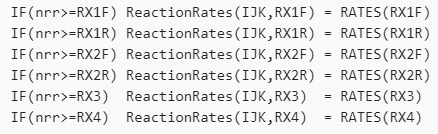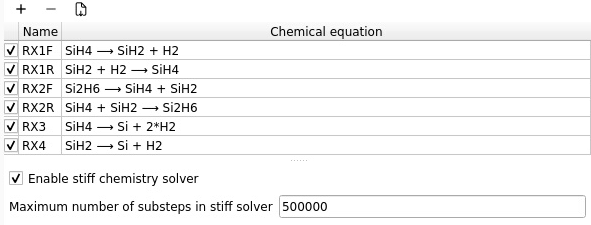# Interface heat transfer coefficient TFM

How can I calculate the interface heat transfer coefficient between the gas and granular phase (2D TFM)?
If I want to add the user define function then where do I have to add this?

Are you familiar with the MFiX keyword browser? Click the magnifying glass at the top of the main screen to activate it. Then entering “Thermal” and clicking “Locate control” takes me to the thermal conductivity control

Yes, I got it, but how to upload the UDF in a particular location? which language file is acceptable. Like, the fluent C+ code language file is acceptable.
My next query is how to calculate the interface heat transfer coefficient, can I use the Costem equation and default any mechanism in the Mfix (Gunn equation)?

MFiX is written in Fortran and UDFs are all in Fortran. (It might be possible to use C but this is not supported by default and you’d have to figure out on your own how to get the code to compile and link.) I suggest you start by studying one of the tutorials that uses UDFs such as Absorption_column_2d.

I did not find the tutorials that use UDFs such as Absorption_column_2d in the Mfix Advance tutorial manual. could you please send me, also send the UDF code file. If possible can you send the one of the case file of TFM which is used the UDF.

Go to New Project and you will be able to load the Absorption column from there:

Dear Friends,
I did not get the solution to the first question, How can I get the interface heat transfer coefficient between the gas and the solid phase?
As I applied the energy equation I got the temperature of both solid and gas phases but then how can I get the heat transfer coefficient that is involved between them?
hsg= (Nu*k_g)/d_p

Sorry, your question is not clear and you have not attached your project files. What thermal conductivity model are you using? Are you trying to specify the effective conductivity, or compute it?

The thermal conductivity model can be changed by UDF but if thermal conductivity is constant then how can I calculate the interface heat transfer coefficient. Here, I attached an equation picture marked in red color which is given in the Mfix manual. How can I get the interface heat transfer when applying the energy equation? please let me know.

This is done in `model/calc_gama.f`

Yes, I saw in the thermochemical cal_gama.f in the model. It means it is running interface. I want to plot the heat transfer coefficient along the axial height so i want the heat transfer coefficient value then how can i get in the Mfix. could you suggest me how can i check in the mfix.

Dear Jeff, and cgw
please let me know the how can I get the heat transfer coefficient between the interface gas and solid phase after the simulation. I checked it in the different sources but I did not find the solution.

You can use the array `ReactionRates(IJK, R)` to save any cell data. It is a legacy array that was used primarily to output reaction rates, but it can be used for other cell data. Here `IJK` is a cell index and `R` is the variable index. This is used along with `nRR`.

Let’s say you want to output 2 variables. First you set `nRR=2` (Model pane> ReactionRates array size).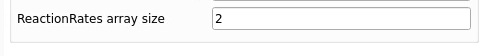Then in any subroutine you want to save the data, add

``````USE rxns, only: Reactionrates
``````

above the variable declaration so `Reactionrates` is available.

In an IJK loop, you can save the two variables:

``````Reactionrates(IJK,1) = ABC
Reactionrates(IJK,2) = DEF
``````

(Replace `ABC` and `DEF` with whatever data you want to save)

To save the data in vtk files, set up a cell data vtk region and go to the 'Reactions` tab, you can select the two reaction rates arrays and provide a label (name) that is representative of the data.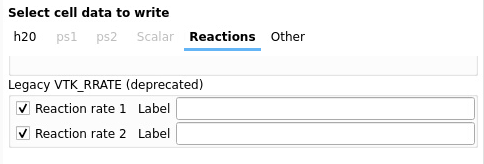1 Like

Here I understood how many reaction rates are applied and stored the result data from the Vtk file. I have still doubts about interface heat transfer hsg. how can I define here and calculate it and how solver identify hsg in reaction rate at 1?
if we are applying 10 reaction rates array size then what is the meaning of 1, 2…10.
how can I identify which reaction is working in 1…10 and if we want to define the interface heat transfer coefficient then how do we identify this?
In the model tab, I applied it but did not understand how to interface the heat transfer coefficient define and calculate it.

Dear Jeff.
I still have doubts about how I can get the data interface heat transfer coefficient (gamma ) during the simulation.
Do i have to define the reaction rates array size =1
and VTK_RRATE name will be gamma
Do I write?
or other we have to define. please let me know.

Please see attached for an example. I took the silane pyrolysis setup and saved gama. This one already has 6 ReactionRates so I saved the data in the seventh slot.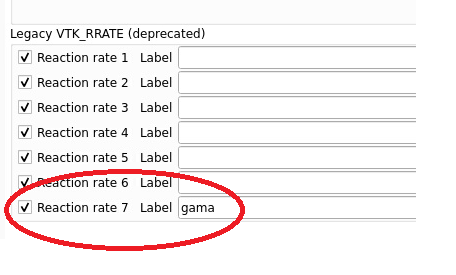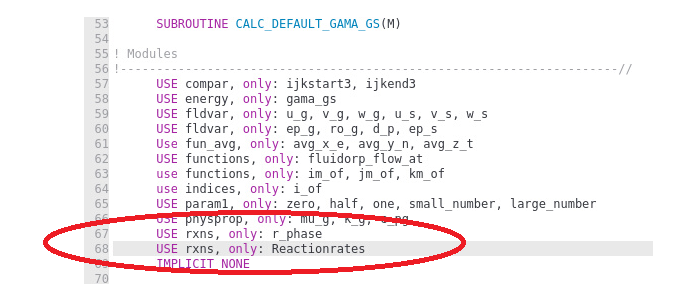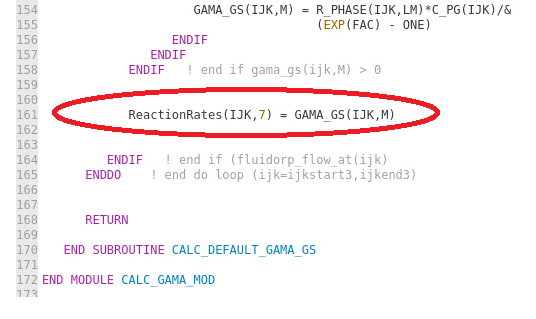Thank you so much for the help. I understand how can i get the data.
I still have some doubts:
why do we define the gamma reaction rate as 7 numbers, we can put it in another number. is this required to define the same order or not?
what is the name of the other reaction, I mean 1 to 6 or more than 7.
can I define the other parameter such as Nu, Re, that is also include the heat transfer transfer equation?

I used the 7th slot because the first 6 were already in use. The order is arbitrary. The other ones are not specifically labeled, these are the 6 reaction rates defines in `usr_rates.f`
You can save more data following the same guidelines, but you must be in an IJK cell loop.

Thanks, Jeff,
I find 6 usr_rates, but I do not understand the meaning of it. I found it in the manual but there is no define for it. I want to know the names of these reactions.